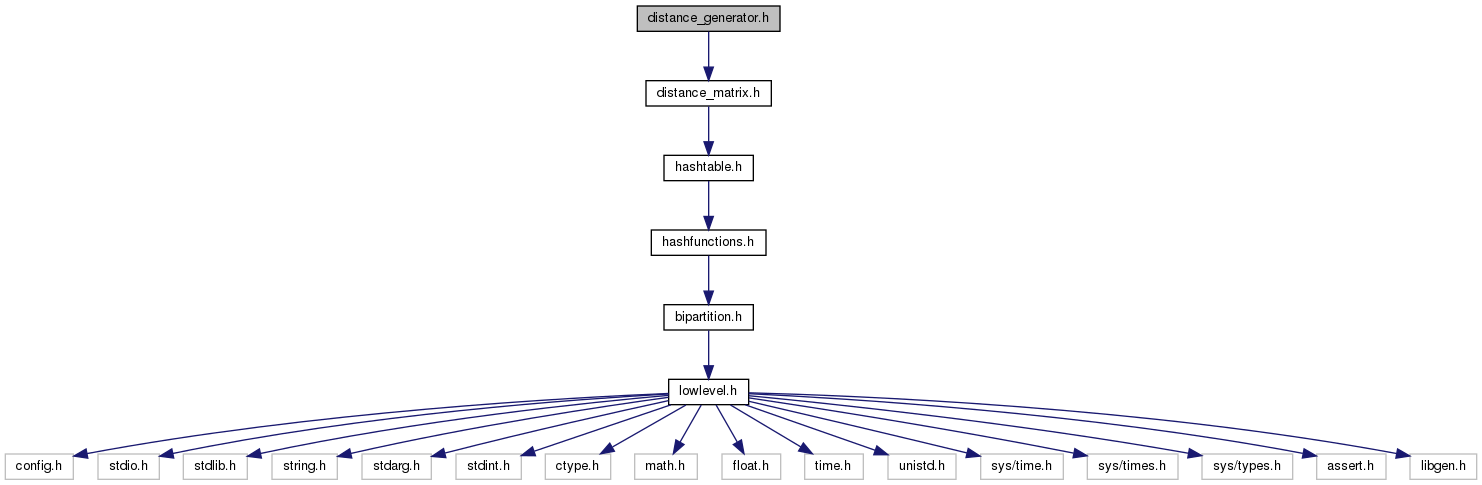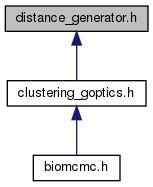biomcmc-lib  0.1 low level library for phylogenetic analysis
distance_generator.h File Reference

distance calculation between generic objects,without generating full matrix beforehand More...

`#include "distance_matrix.h"`
Include dependency graph for distance_generator.h:This graph shows which files directly or indirectly include this file:Go to the source code of this file.

## Data Structures

struct  distance_generator_struct

## Typedefs

typedef struct distance_generator_structdistance_generator

## Functions

distance_generator new_distance_generator (int n_samples, int n_distances)

void del_distance_generator (distance_generator d)

double distance_generator_get_at_distance (distance_generator d, int i, int j, int which_distance)

double distance_generator_get (distance_generator d, int i, int j)

void distance_generator_set_function_data (distance_generator d, void(*lowlevel_dist_funct)(void *, int, int, double *), void *extra_data)
defines distance calculation function wrapper, and all extra data needed by wrapper; no check is done here, but wrapper should return at least as many distances sd n_distances (wrapper functions can check)

void distance_generator_set_which_distance (distance_generator d, int which_distance)
distance wrapper may return several distances, but only one is returned by get(); this sets which one (should be called before e.g. clustering)

void distance_generator_reset (distance_generator d)

## Detailed Description

distance calculation between generic objects,without generating full matrix beforehand